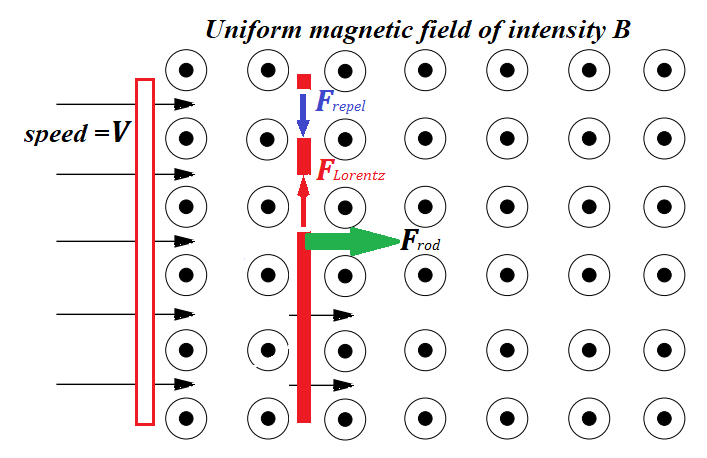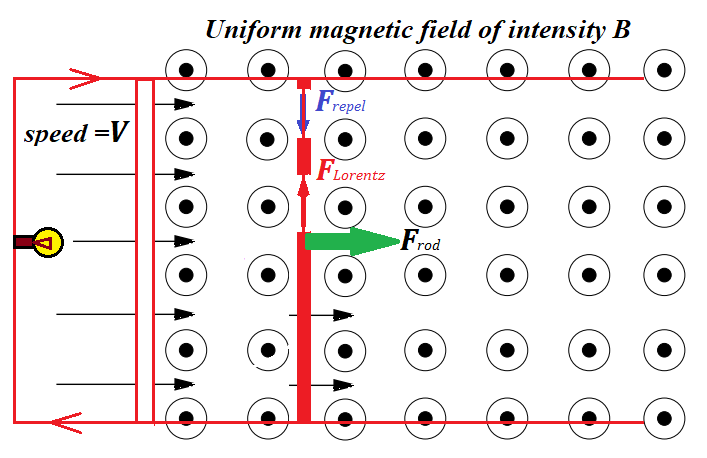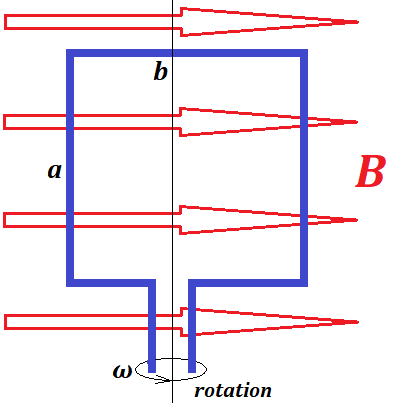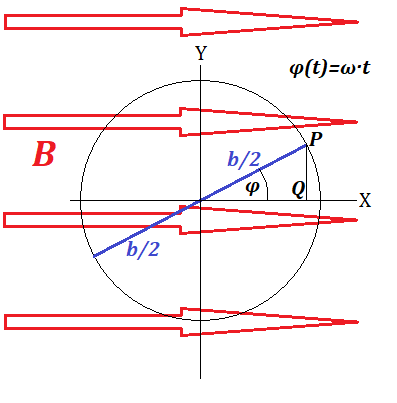## Tuesday, June 23, 2020

### UNIZOR.COM - Physics4Teens - Electromagnetism - The Laws of Induction - Wire Frame Rotation

Notes to a video lecture on http://www.unizor.com

Wire Frame Rotation

Let's start with a summary of our knowledge about electromagnetic induction before embarking on a rotation of a wire frame in a magnetic field.

The foundation of electromagnetic induction is the Lorentz force that is exerted by a magnetic field onto an electrically charged particle moving across the magnetic field lines.As we demonstrated in previous lectures, this force equals to
F = q·V·B
where
q is the particle's electric charge,
V is the speed of this charge in a direction perpendicular to magnetic field lines (if it's not perpendicular, it should be multiplied by sin(φ), where φ is an angle between a velocity vector of a particle and magnetic field lines),
B is the intensity of a magnetic field.
In vector form, considering general direction of a particle's velocity and magnetic field intensity vector, the formula is
F =q·V B
The important fact about the Lorentz force, acting on an electrically charged moving particle (like an electron) is that it acts perpendicularly to both velocity of a particle and the magnetic field intensity vectors.

When we move a metal rod parallel to itself and perpendicularly to magnetic field lines, "free" electrons are pulled by this motion towards the general direction of a rod (green arrow on a picture below).This movement of electrons is perpendicular to magnetic field lines and, therefore, is a subject to Lorentz force that pushes electrons perpendicularly to a general direction of a rod's movement and perpendicularly to magnetic field lines, that is electrons are pushed along the rod to its edge (red vertical arrow on a picture above).

This generates the difference of electric potential on the opposite ends of a rod, one will be positive, another - negative. The faster we move the rod - the stronger Lorentz force is - the greater difference of electric potential will be generated.

The Lorentz force that pushes the electrons to the end of a moving rod meets the resistance of electrostatic forces that repel electrons from each other (blue vertical arrow on a picture above). That's why the concentration of "free" electrons at one end of a rod that moves with certain speed reaches its maximum and stays this way. For every speed of movement of a rod and a particular magnetic field intensity there is a specific difference of potentials or electromotive force (EMF) developed at the ends of a rod.

Let's connect the ends of our rod, that accumulated positive and negative charges as a result of the Lorentz force on moving "free" electrons, through any consumer of electricity, like a lamp.The accumulated excess of electrons from one end of a rod will go to the connecting wire to a lamp and to the positively charged end of a rod. This will diminish a concentration of electrons on a negative end of a rod. While a rod continues its movement across the magnetic field, the Lorentz force acting on "free" electrons inside a rod will push more electrons to the end to replace those that went through a lamp to a positive end of a wire, thus establishing a constant electric current in an electric circuit, generated by mechanical movement of a rod perpendicularly to magnetic field lines.

From the first glance it looks like movement of a rod with constant speed, which does not require any energy to spend, generates electricity and, therefore, free energy, which contradicts the Law of Conservation of Energy. What do we miss?

Let's make one more step in our analysis of this experiment. The electric current generated by a rod's movement runs around a circuit and, therefore, runs through a rod as well perpendicularly to magnetic field lines. As we know, the wire with electric current perpendicular to magnetic field lines experiences another manifestation of the Lorentz force - the one that pushes the whole rod perpendicularly to its length.

The Lorentz force analyzed first acts on electrons moving with a rod to the right on the picture above and pushes them to one end of a rod (upwards on the picture). This creates an electric current in a rod, and the Lorentz force, acting on electrons moving along the rod pushes them and the whole rod with them to the left against the initial movement of a rod, thus forcing the need for some outside force to move the rod with constant speed.

As we see, a magnetic field acts against the movement of a rod.
It means, to move a rod, we have to overcome the resistance and perform some work. This work, according to the Law of Energy Conservation, is converted in some other type of energy, like into heat in the lamp on a circuit.

If we follow the trajectory of "free" electrons inside a rod, we would see that they move simultaneously in two directions - to the right with rod's movement and upwards because of the Lorentz force acting on moving particles in a magnetic field perpendicularly to the field's intensity vector.

In vector form the velocity vector of an electron can be represented as
Ve = Vr + Vu
where
Ve is velocity of an electron,
Vr is its velocity to the right,
Vu is its velocity upwards.

Therefore, the total Lorentz force is
F =q·(Vr +Vu ) B   =
= q·Vr
B + q·Vu B   =
= Fu + Fl

where
Fu  is upward component of the Lorentz force that pushes electrons towards the end of a rod,
Fl   is its component to the left against the rod's motion.

The electromotive force (EMF) generated by the movement of a rod was quantitatively evaluated in the previous lecture. As the rod moves to the right, thus increasing the length of two sides of a circuit's rectangular frame, the magnitude of a generated EMF was equal to the rate of a change of a magnetic field flux through the frame:
|U| = |dΦ/dt|
where the magnetic field flux was defined as a product of magnetic field intensity B and the area of a wire frame evaluated in a direction perpendicular to the magnetic field lines.
This is the Faraday's Law of electromagnetic induction.

To better understand a concept of magnetic field flux we can use a concept of the "number" of magnetic field lines. These lines point toward the direction of magnetic field forces and their density represents the strength of these forces. Using this concept, magnetic field flux is analogous to the "number" of magnetic field lines going through a wire frame. Wider wire frame, more intense magnetic field - greater magnetic field flux goes through the wire frame.

That concludes the summary of our knowledge about electromagnetic induction, as presented so far, and we are ready to switch to a more practical way of generating the electricity from mechanical movement - from a rotation of a wire frame.

Considering it's impractical to change the magnetic flux by changing the geometry of a wire frame, a different approach to generate electricity was suggested - to rotate a wire frame. Rotating it, we change the angle between the wire frame plane and the magnetic field lines, thus changing the magnetic flux going through a frame.
Let's examine closer this process.

Consider a rotation of a wire frame with dimensions a by b, rotating with a constant angular speed ω in the uniform magnetic field B.Consider the initial position of a wire frame to be, as shown on a picture above, with an axis of rotation perpendicular to magnetic field lines and side b of a frame parallel to magnetic field lines. Then side a would be perpendicular to magnetic field lines.
As the frame rotates with an angular speed ω, the angle of a side b with the lines of a magnetic field, as a function of time, is
φ(t) = ω·t

Side b of a wire frame and its opposite do not sweep across magnetic field lines, only side a and its opposite do.
Side a and its opposite are crossing magnetic field lines in opposite directions. Viewing in the direction of the magnetic field lines, if side a crosses the magnetic field lines from right to left, the opposite side at the same time crosses these magnetic lines left to right.

As the wire frame rotates, the "free" electrons inside side a and its opposite are pushed by the rotation of a wire across the magnetic field lines in opposite directions and, therefore, are pushed by the Lorentz force, exerted by a magnetic field on these electrons, towards opposite ends of corresponding sides of wire frame. If electrons in side a are pushed upwards, the electrons in the opposite side are pushed downwards and vice versa.
As a result, there is an electric current generated in a rotating wire frame.

When a wire crosses the magnetic field lines with "free" electrons inside, Lorentz force on electrons inside the wire is proportional to a speed of a wire across the magnetic field lines in a direction perpendicular to these lines. Consequently, the faster a wire is crossing the magnetic field lines in a perpendicular to them direction - the more electric charge inside a wire is separated between positive and negative ends. Therefore, the electric current generated by the movement of a wire across the magnetic field lines is proportional to a speed of perpendicularly crossing these lines.

At its original position at time t=0 side a crosses more magnetic field lines per unit of time than at position of a wire frame turned by 90°. The "number" of magnetic field lines crossed per unit of time is maximum at angle φ(t)=0 and minimum (actually, zero) at φ(t)=π/2.
As the wire frame continues its rotation, the "number" of magnetic field lines crossed per unit of time increases and reaches another maximum at φ(t)=π, then diminishes to zero at φ(t)=3π/2.

To calculate the speed of crossing the magnetic field lines in a perpendicular to them direction by sides a and its opposite, consider a top view onto our wire frame.Side a and its opposite are circulating around the axis of rotation on a radius b/2. During time t the angle of rotation will be φ(t)=ω·t.

The speed of crossing the magnetic field lines by side a or its opposite is a Y-component of a velocity vector of the points P or its opposite.

The Y-coordinate of point P is
PQ = b·sin(φ)/2 = b·sin(ω·t)/2.
Therefore, the Y-component of a vector of velocity of point P is a derivative of this function
VY = b·ω·cos(ω·t)/2.

Analyzing this expression, we quantitatively confirm our considerations about speed of crossing the magnetic field lines presented above.
At t=0 this function is at maximum and equals to b·ω/2 - linear speed of a point-object rotating with angular speed ω on a radius b/2.
At t=90° the value of VY is zero because the point P moves parallel to X-axis.
Than the Y-component of velocity goes to negative part, crossing the magnetic field lines in an opposite direction etc.
During one full rotation the direction of an electric current in a wire frame will change because each side half of a circle crosses the magnetic field lines in one direction and on another half of a circle crosses them in an opposite direction. This is how alternate current is produced by electric power plants.

As Lorentz force on "free" electrons within side a separates them from their atoms and pushes to one end of this side, Lorentz force on the opposite side of a frame performs similar action, separating electrons towards the opposite end of a wire, thus assuring the electrical current inside a wire frame I=I(t).

Rotation of side a and its opposite can be represented as simultaneous movement along two coordinates. Movement along X-axis, as presented on a picture above, does not cross magnetic field lines, there is no Lorentz force related to this movement, no separation of electrons from their nuclei, no EMF.
But movement along Y-axis does cross magnetic field lines and, therefore, the Lorentz force pushes electrons to one end of a wire and resists the rotation by acting against the movement along the Y-axis.
As we have an electric current I(t) inside a wire frame, there is a Lorentz force acting upon side a and its opposite that needs to be overcome to assure the rotation with constant angular speed ω.

The force on side a directed against its movement along the Y-axis is
Fa(t) = I(t)·a·B
and the same by magnitude force, but acting upon a side opposite to a, also against its movement along Y-axis.
Both these forces are acting against the rotation. When side a moves in the positive direction of the Y-axis, the force Fa(t) is directed towards the negative one and vice versa. Same with the side opposite to side a.

The key consideration now is the following.
The force Fa(t) and its opposite must be overcome by a rotation, that is some work must be exerted by a rotating mechanism, which, according to the Law of Energy Conservation, must be equal to amount of work the generated electric current exerts by circulating in the wire frame.
That is, mechanical work of rotation is converted into electrical work - electric power is generated by a rotation.

The Y-coordinate of point P, rotating around the origin of coordinates with an angular speed ω on a radius b/2, as a function of time t, is
PY(t) = b·sin(ω·t)/2
The Y-component of a velocity vector of point P is a derivative of this function
VY(t) = b·ω·cos(ω·t)/2

During the time from t to t+dt point P and, therefore, side a will be moved by a rotation in the Y-direction by a distance
dSY = VY(t)·dt =
= b·ω·cos(ω·t)·
dt/2

Therefore, the work needed to overcome the force Fa(t), acting in the direction of Y-axis against the rotation of side a, during the time from t to t+dt is
dWa = Fa(t)·VY(t)·dt =
=
[I(t)·a·B]·b·ω·cos(ω·t)·dt/2

The same by magnitude and opposite in direction force acts on a side opposite to a, also acting against its movement in the direction of the Y-axis. Therefore, the increment of work needed to be exerted by a rotation mechanism to assure the rotation at angular speed ω, as a function of time t during the time from t to t+dt is
dW = [I(t)·a·B]·b·ω·cos(ω·t)·dt

The power exerted by a rotating mechanism at time t is
P(t) = dW/dt =
= I(t)·a·B·b·ω·cos(ω·t)

During this period from t to t+dt the electricity generated by the rotation of the wire has the value of current equal to I(t). The cause of this current is the electromotive force (EMF) or, simply, voltage U(t) generated by Lorentz force on "free" electrons that separates them from their atoms.

The power exerted by an electric current I with voltage U is P=I·U.
Equating this power of electric current to a power exerted by a rotating mechanism, as the Law of Energy Conservation dictates, we obtain
I(t)·a·B·b·ω·cos(ω·t) = I(t)·U(t)
From this we can find EMF generated by a rotation of a wire frame:
U(t) = B·a·b·ω·cos(ω·t)
Applying simple geometry, we can see that the area of a wire frame perpendicular to magnetic lines at time t equals to
S(t) = a·b·sin(ω·t)
Therefore, magnetic field flux through the wire frame at time t is
Φ(t) = B·a·b·sin(ω·t)
The rate of change of this flux is, therefore, a derivative
dΦ(t)/dt = B·a·b·ω·cos(ω·t)

Comparing this with the above expression for EMF, we see that
U(t) = dΦ(t)/dt
which is the same as in a case of a rod moving parallel to itself on rails perpendicularly to the magnetic field lines, discussed in the prior lecture.
This brings us to an important generalization of the Faraday's Law.
If the magnetic flux going through a wire loop is changing, the rate of this change equals to an electromotive force generated inside a wire.